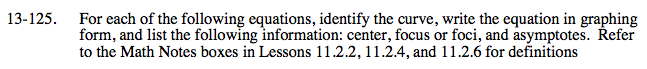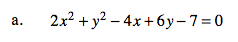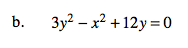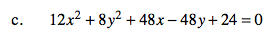### Home > A2C > Chapter 13 > Lesson 13.2.2 > Problem13-125

13-125.$\frac{(x-1)^2}{9}+\frac{(y+3)^2}{18}=1$

Center: (1, − 3)
Foci: (1, 0) and (1, −6)$\frac{(y+2)^2}{4}-\frac{x^2}{12}=1$

Now find the center, foci and asymptotes.See parts (a) and (b).